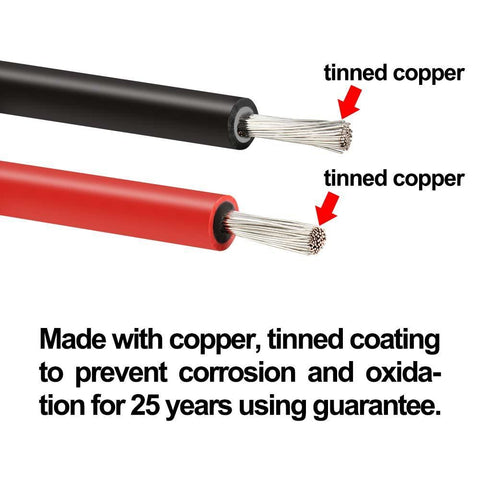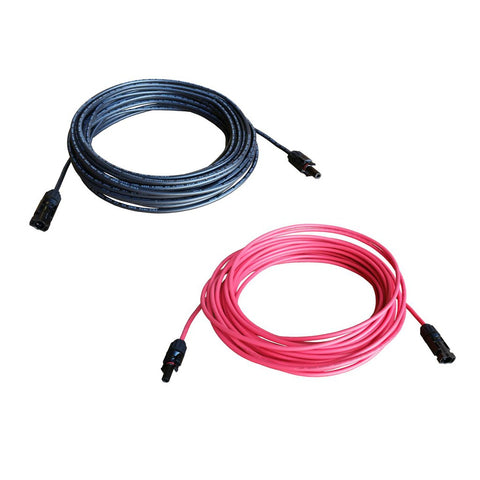What Gauge Wire For 100 Watt Solar Panel - ShopSolar.com

# What Gauge Wire for 100 Watt Solar PanelYou might not think so, but the gauge of the wires you use for your solar panel array plays a crucial role in the running of your solar system.

It may seem like “just a little wire”, but correctly sized wires are imperative for not only the performance of your solar array but for your own safety, too.

In this article, we will be discussing how to calculate what size wire gauge you need for your specific solar array, the reason why the wire size is so important, as well as what gauge of wire is most appropriate for a 100-watt solar panel.## How to Calculate the Wire Gauge Needed for a 100-Watt Solar Panel

When calculating wire gauge, there is not necessarily a “one size fits all” for 100-watt solar panels. The wire gauge needs to be calculated in accordance with your solar array.

You will have to look at your 100-watt solar panel specifications, figure out what size battery for a 100 watt solar panel, and look at the requirements of your array as a whole to do so.

### Different Ways to Calculate Wire Gauge

There are various different ways that you can calculate what wire gauge you will need for your 100-watt solar panel.

The easiest way to calculate it is by using an online calculator made specifically for wire gauge estimation. All you need to do is input certain variables, that we will speak about shortly, into a calculator and it provides an estimate almost instantaneously.

Another way to calculate wire gauge is through a manual equation. This is more tedious, but we will go through this method too, in case it is your preferred option.

Despite the option used to calculate wire gauge, the same variable will be used in both.

Below we will take you through what those variables are.

### Variables and Parameters to Focus on When Calculating Wire Gauge

Three main variables need to be considered when calculating the wire gauge required for your solar array.

These variables include the voltage of the system, the maximum amperage that will be produced by your solar panel, and the distance between the batteries and the solar panels, or between the batteries and charge controller.

Different wire gauges can only handle a certain amount of voltage and amperage, which is why it is important to look at the specifications of your solar panel as your starting point.

If you look at the Inergy Linx 100 Watt Flexible Solar Panel, available from Shop Solar Kits, you will see that the voltage at maximum power (Vm) is 17.6V, and the current at maximum power (Im) is 5.68A.

This means that our gauge wire needs to be big enough to safely handle these two measurements.### Manually Calculating Wire Gauge for a 100 Watt Solar Panel

As an example, we will calculate the wire gauge needed for the wire that runs between a single 100-watt solar panel and a 12V battery bank.

We will be working with the Inergy Linx 100 Watt Flexible Solar Panel, available from Shop Solar Kits. This solar panel has a voltage at the maximum power of 17.6V, and a current at the maximum power of 5.68A.

To work out the target resistance, we will use ohm’s law which states that V = IR. This means that 17.6/5.68 = 3.09 ohms, which is the resistance that will occur for this solar panel.

We are going to assume that the cable you are purchasing is made out of copper, as that is the most common material for solar panel wiring.

Copper has a resistivity of 1.724 x 10^-8 ohm meters at room temperature, and you will have to multiply this by the meters that the wire needs to be. In this case, we will say that it has to be at least 50 meters long.

50 x 1.724 x 10^-8 = 8.62 x 10^-7 ohm square meters.

Next, we get the wire’s cross-sectional area by dividing our answer by our target resistance.

8.62 x 10^-7/3.09 = 2.79^-7.

To find the wire’s radius in meters, we will first have to divide our above answer by pi.

2.79^-7/3.142 = 8.88^-8 meters.

We then need to find the square root of this answer, which will be 0.000297. To get this radius into inches, we will multiply it by 39.37.

0.000297 x 39.37 = 0.012 inches.

This then needs to be multiplied by 2 in order to get the diameter of the wire, so our end answer would be 0.024 inches. This approximately equates to a 21 AWG.

As you can see, the wire gauge for a 100-watt solar panel can be calculated manually, but it is an extremely tedious process, and there is a lot of room for human error due to the complex numbers that are involved.

For the same 100-watt solar panel, we know that it has a maximum current of 5.68 A.

If you look at the capacity row, 14 AWG is the smallest wire you can use to support this.

Now, if you look at the “array amps” column, we will look at 6 amps, as this is the closest choice to 5.68. If you match up the 6 amps with the 14 AWG, we see that it can safely cover 7.5 feet, which isn’t very far.

So, if we bump it up to a 10 or even 4 AWG, we can have a much longer wire.

But, as you can see, the two different manual calculations gave very different gauges for the same solar panel, which makes this method quite risky.

To save time and improve accuracy, we would recommend using a wire gauge calculator that can be found online.## What is a Gauge Wire?

For those who are unsure, the gauge of your wire is also known as the diameter of the wire. The diameter of copper wires is sized according to a gauge scale known as American Wire Gauge (AWG).

Gauge wire determines how much electrical current can be safely carried through the wire without causing damage to the wire, or potentially starting an electric fire

The wire gauge also determines the resistance of the wire, as well as its weight per unit length.

In terms of sizing, the AWG numerical value is inversely proportional to the conductor’s diameter. In other words, if the gauge number is lower, it means that the diameter of the wire is bigger, and, as a result, there will be less resistance to the flow of current.

The same is true for the opposite circumstance - a bigger AWG equates to a small diameter, which results in more resistance to current.

Generally speaking, a bigger diameter is safer because it is usually safer due to the minimal resistance. However, it is also normally more expensive, so it is important to find the sweet spot of what wire is big and safe enough, but also won’t break the bank.

It is important to note that the AWG value does not account for the insulator covering the wire. It is simply a measurement of the diameter, or cross-sectional area, of the wire itself.

## The Importance of Using Correctly Sized Wire in a Solar Panel Array

The wire you use in your solar panel system may seem like an unimportant, or nonurgent, afterthought.

However, figuring out the correct size of wire you need is just as important as finding the answers to questions like “What size charge controller do I need for a 100-watt solar panel?” and “What size inverter do I need for a 100-watt solar panel?”

When we refer to the “size” of the wire, we are referring to the length, as well as the gauge of the wire.

### Why Does the Size Make Such a Difference to the Operating of the Solar Array?

The reason these two aspects are so important is that when the gauge of the wire is larger, it will offer less resistance to the flow of current. If the wire’s gauge is small, there will be a lot more resistance to flow.

The length of the wire also affects the resistance to flow. Longer wires pose a larger resistance to the flow of current than shorter ones with the same diameter do.### Why Is It Necessary to Minimize Resistance to Current Flow?

The reason that we want to minimize how much resistance is created by the wire is for two reasons.

Firstly, if the wire is undersized and too much resistance is created, this will lead to a large drop in the voltage of the wires, and this will result in an immense loss of power in the system.

This loss in power will not only lead to a decrease in the performance of your solar array but may also end up costing you money due to how much power is being lost.

Secondly, undersized wires can be very dangerous. If the diameter is too small, it can lead to the wire heating up beyond what it can handle, and can possibly start a fire.

This is a danger to your safety, as well as the safety of your solar system.

## Voltage Drop

Something that is very important to note and consider is the voltage drop of the wire.

The voltage drop is the amount of voltage that is lost along the wire due to resistance. If the wire is undersized, the voltage drop will be massive.

This is something that you don’t want, as it leads to you wasting a large amount of electricity. This is also something that wasn’t factored into the first manual calculation, leaving more room for error.

Voltage drop is especially important to calculate on wires longer than 50 feet, and it is recommended to try to keep it below 3-5%.

## Connecting Your Solar Panels in Series vs Parallel

If you are planning on using more than one solar panel, knowing how to connect 2 100 watt solar panels, and whether you connect them in series or parallel makes a difference for your wire gauge.

For solar panels that are connected in series, you get a higher voltage and a lower amperage. All the panels connected in series are basically seen as one solar panel - they all have the same voltage, amps, and gauge wire.

In parallel, it is the opposite. The voltage stays the same, but the amperage is added together, as each panel acts as an individual, and they don’t “form” one.

The way they are connected will affect the gauge wire you need, so if you are unsure how to install 100-watt solar panels, and what would be the best way to do so, try and get someone to help you and offer you advice as to what would be best for your solar array.

### Final Thoughts

Although it might be assumed that the wire isn’t an important part of your solar array, you can see how it, and especially its gauge and length, play such a crucial role in your system.

Take the time to figure out what gauge size you need, and rather spend a little bit extra money on it to ensure that the quality is top-notch.

This could save you a lot of money and time in the long run and could essentially save your life if it prevents a fire from occurring.

Did you find our blog helpful? Then consider checking: